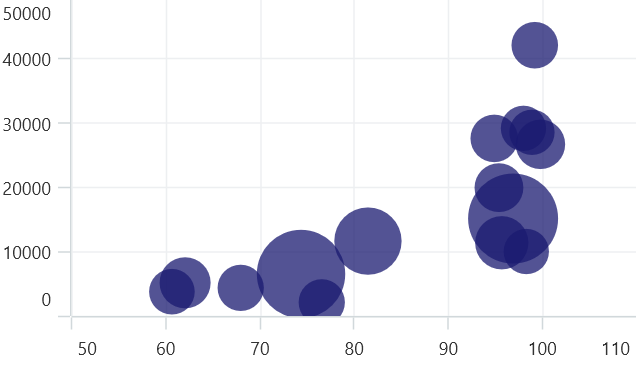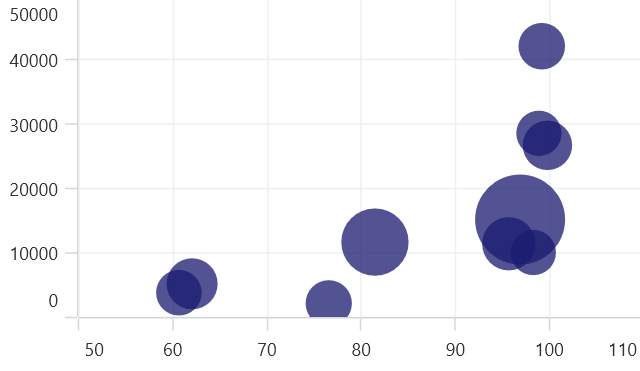# Bubble Chart in .NET MAUI Chart

27 Mar 20234 minutes to read

The bubble chart is represented by closely packed circles, whose areas are proportional to the quantities.

## Bubble Chart

To render a bubble chart, create an instance of BubbleSeries, and add it to the Series collection property of SfCartesianChart. The bubble chart is similar to a scatter plot, but with the additional dimension of bubble size to represent the third variable.

The size of the BubbleSeries is relatively proportional to the value bound with the series using the SizeValuePath property. You can set the constraints on this size using the MinimumRadius and MaximumRadius. The following code illustrates how to set the value to the property.

NOTE

The Cartesian chart has Series as its default content.

``````<chart:SfCartesianChart>
<chart:SfCartesianChart.XAxes>
<chart:NumericalAxis />
</chart:SfCartesianChart.XAxes>

<chart:SfCartesianChart.YAxes>
<chart:NumericalAxis />
</chart:SfCartesianChart.YAxes>

<chart:BubbleSeries ItemsSource="{Binding Data}"
XBindingPath="XValue"
YBindingPath="YValue"
SizeValuePath="Size"/>

</chart:SfCartesianChart>``````
``````SfCartesianChart chart = new SfCartesianChart();
NumericalAxis primaryAxis = new NumericalAxis();
NumericalAxis secondaryAxis = new NumericalAxis();

BubbleSeries series = new BubbleSeries()
{
ItemsSource = new ViewModel().Data,
XBindingPath = "XValue",
YBindingPath = "YValue",
SizeValuePath = "Size",
};

this.Content = chart;``````## Show zero size bubbles

The zero size bubble segments can be enabled or disabled using the ShowZeroSizeBubbles property. By default, the property value is True. The following code illustrates how to set the value to the property.

``````<chart:SfCartesianChart>
. . .
<chart:BubbleSeries ItemsSource="{Binding Data}"
XBindingPath="XValue"
YBindingPath="YValue"
SizeValuePath="Size"
ShowZeroSizeBubbles="False"/>

</chart:SfCartesianChart>``````
``````SfCartesianChart chart = new SfCartesianChart();
...

BubbleSeries bubbleSeries = new BubbleSeries()
{
ItemsSource = new ViewModel().Data,
XBindingPath = "XValue",
YBindingPath = "YValue",
SizeValuePath = "Size",
ShowZeroSizeBubbles = false;
};